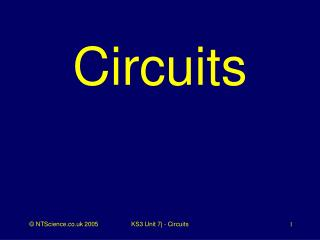DownloadDownload PresentationCircuits

Circuits

Télécharger la présentationCircuits

- - - - - - - - - - - - - - - - - - - - - - - - - - - E N D - - - - - - - - - - - - - - - - - - - - - - - - - - -
Presentation Transcript

1. Circuits KS3 Unit 7j - Circuits

2. Q1. What do you call an electric circuit with components placed one after the other? KS3 Unit 7j - Circuits

3. A1. A Series circuit KS3 Unit 7j - Circuits

4. Q2. What do you call an electric circuit with more than one complete loop? KS3 Unit 7j - Circuits

5. A2. A parallel circuit KS3 Unit 7j - Circuits

6. Q3. What turns chemical potential energy into electrical energy? KS3 Unit 7j - Circuits

7. A3. A cell KS3 Unit 7j - Circuits

8. Q4. What do you call two or more electric cells connected together? KS3 Unit 7j - Circuits

9. A4. Battery KS3 Unit 7j - Circuits

10. Q5. What do you call pieces of metal used to connect electrical components? KS3 Unit 7j - Circuits

11. A5. Wire KS3 Unit 7j - Circuits

12. Q6. What do we use to make or break a complete circuit? KS3 Unit 7j - Circuits

13. A6. A switch KS3 Unit 7j - Circuits

14. Q7. What do you call a thin metal wire that is designed to melt when the electric current is too large.? KS3 Unit 7j - Circuits

15. A7. A fuse KS3 Unit 7j - Circuits

16. Q8. Which electrical component transforms electrical energy into sound energy? KS3 Unit 7j - Circuits

17. A8. Loudspeaker Buzzer KS3 Unit 7j - Circuits

18. Q9. Which electrical component transforms electrical energy into light? KS3 Unit 7j - Circuits

19. A9. Lamp LED KS3 Unit 7j - Circuits

20. Q10 Which battery terminal is usually coloured black? KS3 Unit 7j - Circuits

21. A10. Negative KS3 Unit 7j - Circuits

22. Q11. Which battery terminal is usually coloured red? KS3 Unit 7j - Circuits

23. A11. Positive KS3 Unit 7j - Circuits

24. Q12. Which electrical device measures electric current? KS3 Unit 7j - Circuits

25. A12. Ammeter KS3 Unit 7j - Circuits

26. Q13. Which electrical device measures potential difference (voltage) KS3 Unit 7j - Circuits

27. A13. Voltmeter KS3 Unit 7j - Circuits

28. Q14. Electric current is the flow of what? KS3 Unit 7j - Circuits

29. A14. Electric charge KS3 Unit 7j - Circuits

30. Well Done!Now check your score KS3 Unit 7j - Circuits